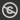Science, Maths & Technology
Free course

# Numbers, units and arithmetic

Free statement of participation on completion## Course reviews

Do fractions and decimals make you apprehensive about maths? Do you lack confidence in dealing with numbers? If so, then this free course, Numbers, units and arithmetic, is for you. The course will explain the basics of working with positive and negative numbers and how to multiply and divide with fractions and decimals.

## Course learning outcomes

After studying this course, you should be able to:

• write whole numbers and decimals in place-value columns and compare their sizes
• multiply and divide whole numbers and decimals by 10, 100, 1000 and so on
• indicate given fractions on a diagram and find equivalent fractions for a given fraction
• mark numbers on a number line
• choose appropriate units for a given purpose.

First Published: 21/12/2012

Updated: 10/05/2019

Skip Rate and Review